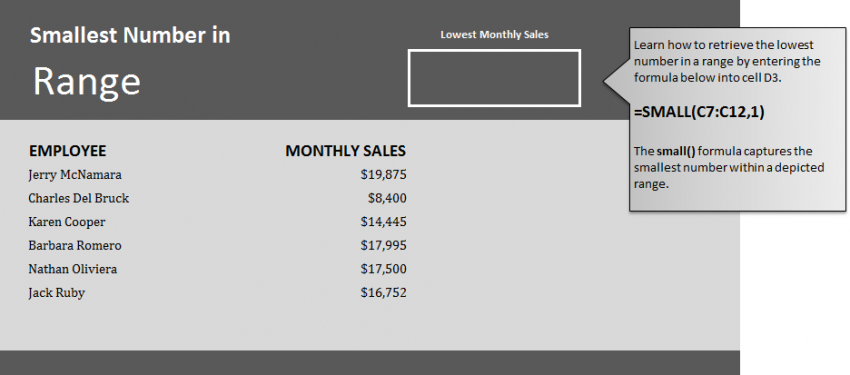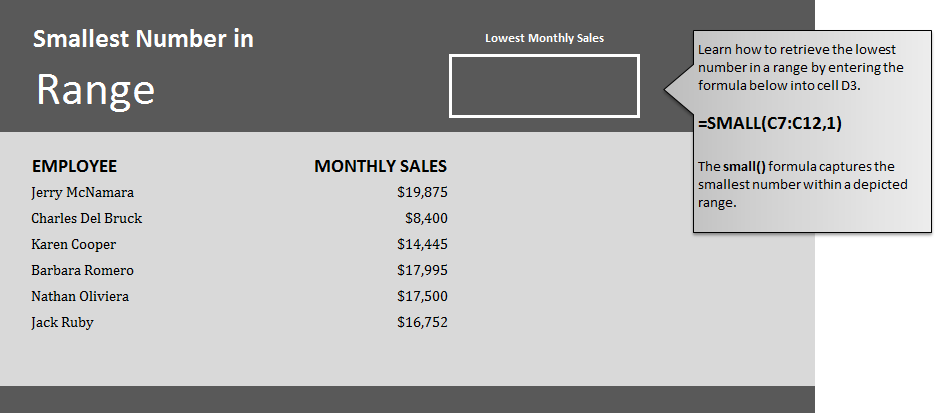# Find Smallest Number in Excel14
AprThis formula is used to find smallest number in Excel spreadsheets. Learn how to find the smallest number in a range within an Excel worksheet. This is useful when calculating grades, percentages, currency amounts, and stats of groups. The formula to get the smallest number in a range is simple and can be a very good tool to have.

Download the example spreadsheet to learn how to use this formula.

Open the worksheet and you will see a list of employees and beside each name, a currency number of their monthly sales numbers. Your goal is to have the formula search the Excel file for the lowest number.

To do this, enter the following formula in cell D3:

=SMALL(C7:C12,1)

The result should show that Charles Del Bruck has the lowest sales at \$8,400.

The formula takes the range of cells you input (C7 to C12) and searches that range for the lowest number. You can adjust these ranges to search just sections of employees to help categorize where your sales team stands.

When using this formula in your own spreadsheet, simply change the cell range you need searched and pick a blank cell to give you the result.